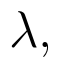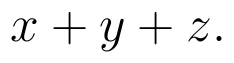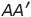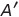Select Page

# 12 Science Maths CBSE Solutions for MCQ Differential Equations in Hindi

12 Science Maths CBSE Solutions for MCQ Differential Equations in Hindi to enable students to get Solutions in a narrative video format for the specific question.

Expert Teacher provides 12 Science Maths CBSE Solutions for MCQ Differential Equations through Video Solutions in Hindi language. This video solution will be useful for students to understand how to write an answer in exam in order to score more marks. This teacher uses a narrative style for a question from Differential Equations not only to explain the proper method of answering question, but deriving right answer too.

Please find the question below and view the Solution in a narrative video format.

Question:

Solution Video in Hindi:

You can select video Solutions from other languages also. Please check Solutions in ( English )

## Similar Questions from CBSE, 12th Science, Maths, Differential Equations

Question 1 : Write the degree of the differential equation :(View Answer Video)

Question 2 : Write the degree of the differential equation :(View Answer Video)

Question 3 : Write the degree of the differential equation(View Answer Video)

Question 4 : Write the sum of the order and degree of the differential equation(View Answer Video)

Question 5 : Find the general solution of differential equation(View Answer Video)

### Inverse Trigonometric Functions

Question 1 :is equal to :

### Vector Algebra

Question 1 : Find a unit vector in the direction of(View Answer Video)

Question 2 : Write the position vector of the point which divides the join of points with position vectorsandin the ratio 2:1.  (View Answer Video)

Question 3 : Find the value ofif the points with position vectorsandare coplanar.  (View Answer Video)

Question 4 :  Ifandare two equal vectors, then write the value of(View Answer Video)

Question 5 :  Write a unit vector in the direction of the sum of vectorsand(View Answer Video)

### Matrices

Question 1 :  Find the value of y, if(View Answer Video)

Question 2 : LetFind A - B. (View Answer Video)

Question 3 : Compute:. (View Answer Video)

Question 4 : If, write, whereis the transpose of A? (View Answer Video)

Question 5 : Find the transpose of the matrix:. (View Answer Video)